# Skew Lines Skew Lines The two given lines

• Slides: 27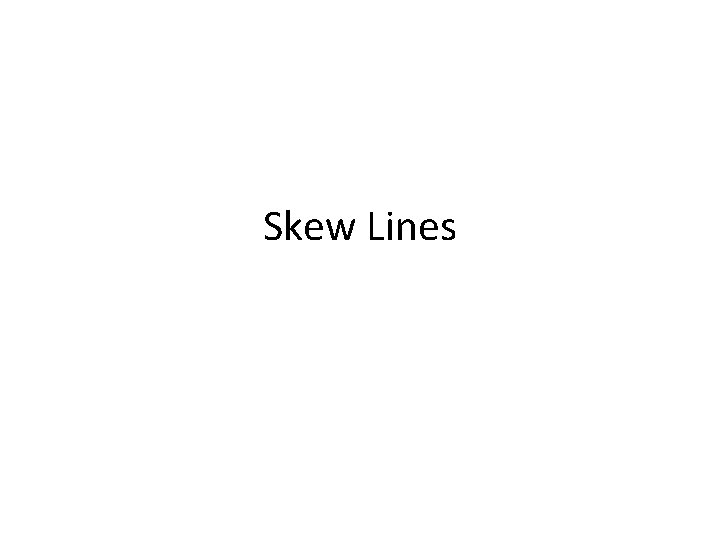Skew LinesSkew Lines The two given lines given are skew (i. e. they do not meet). What is the shortest distance between them? shortest distance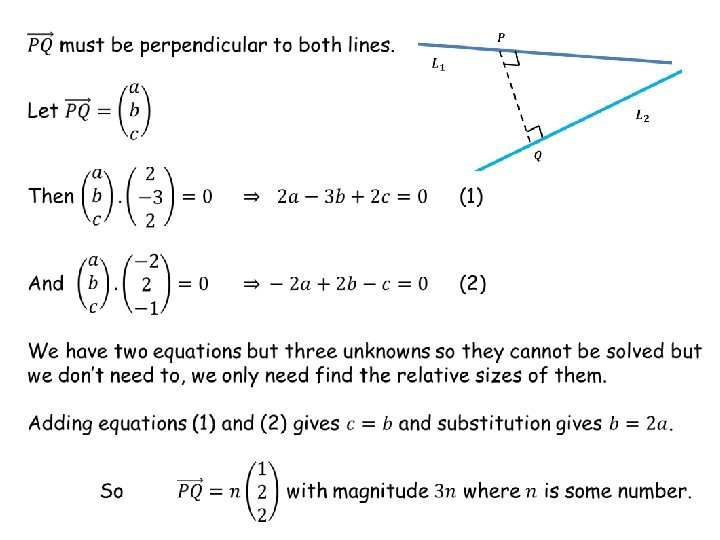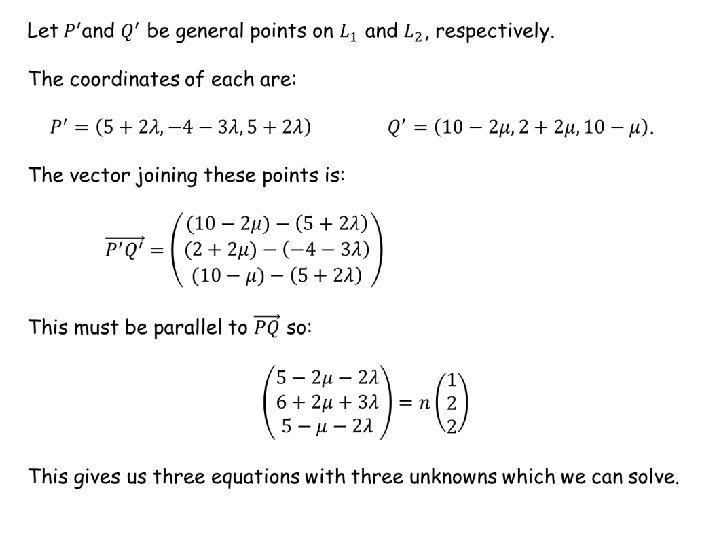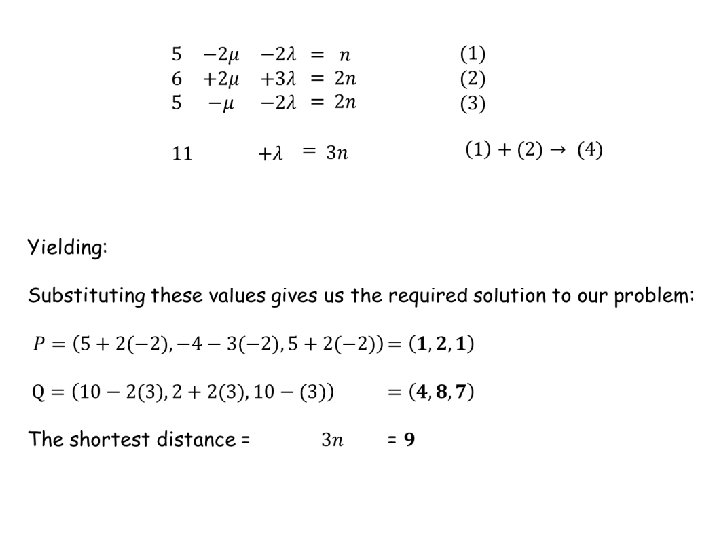RESOURCES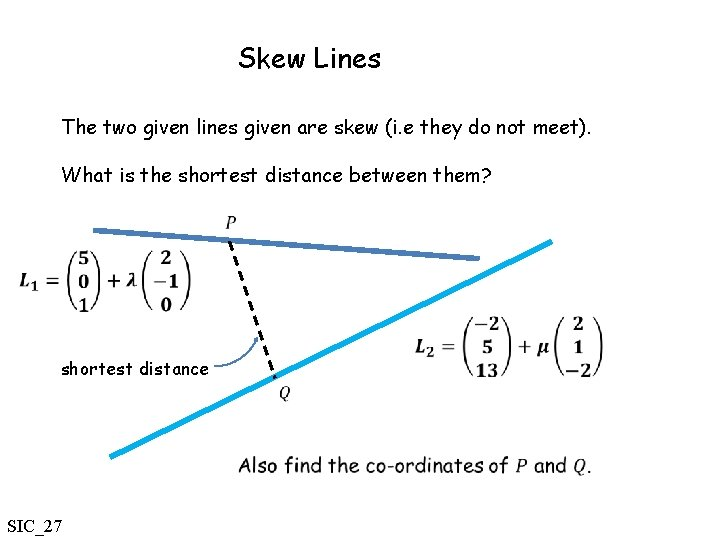Skew Lines The two given lines given are skew (i. e they do not meet). What is the shortest distance between them? shortest distance SIC_27Skew Lines The two given lines given are skew (i. e they do not meet). What is the shortest distance between them? shortest distance SIC_27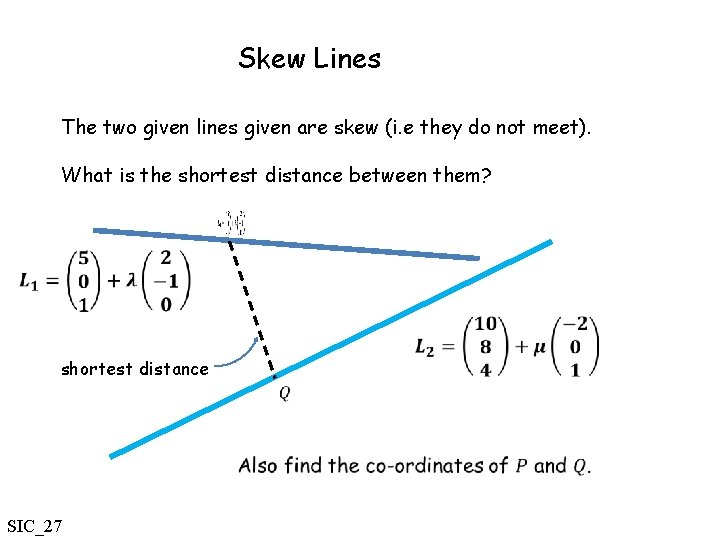Skew Lines The two given lines given are skew (i. e they do not meet). What is the shortest distance between them? shortest distance SIC_27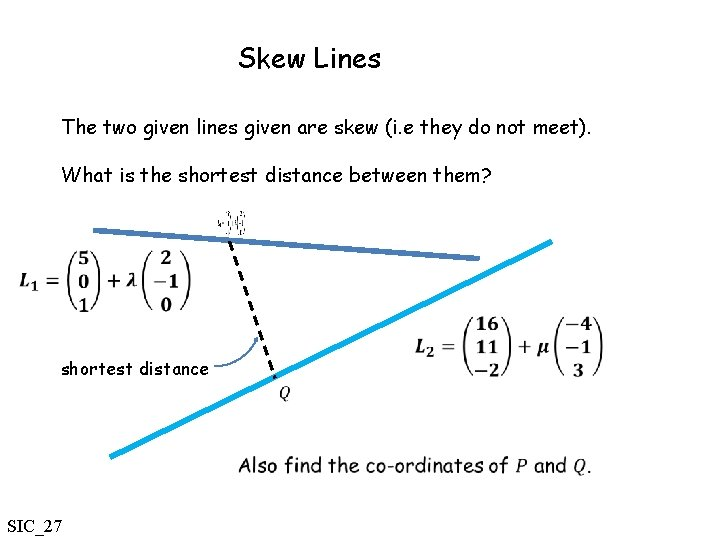Skew Lines The two given lines given are skew (i. e they do not meet). What is the shortest distance between them? shortest distance SIC_27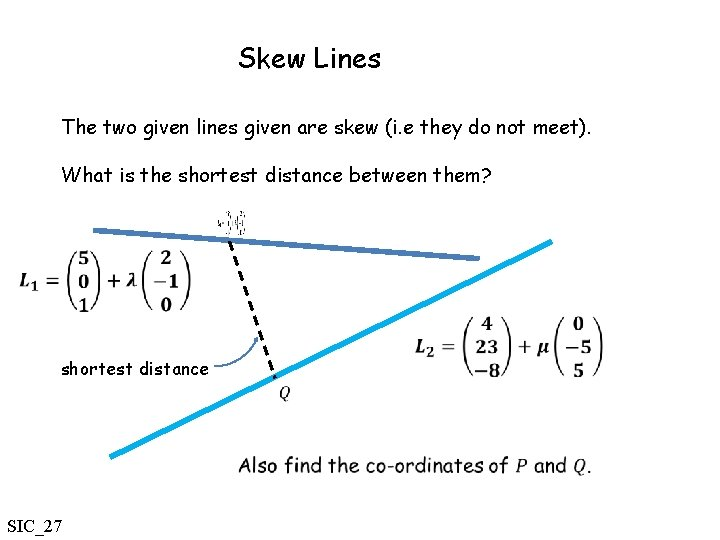Skew Lines The two given lines given are skew (i. e they do not meet). What is the shortest distance between them? shortest distance SIC_27Skew Lines The two given lines given are skew (i. e they do not meet). What is the shortest distance between them? shortest distance SIC_27Skew Lines The two given lines given are skew (i. e they do not meet). What is the shortest distance between them? shortest distance SIC_27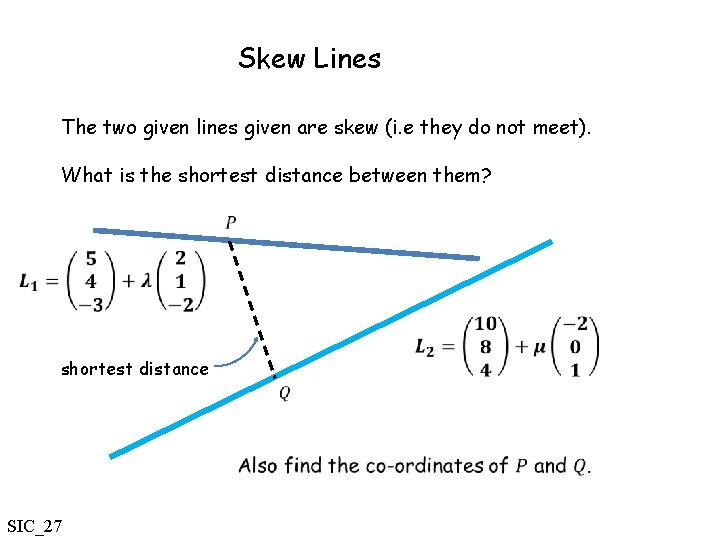Skew Lines The two given lines given are skew (i. e they do not meet). What is the shortest distance between them? shortest distance SIC_27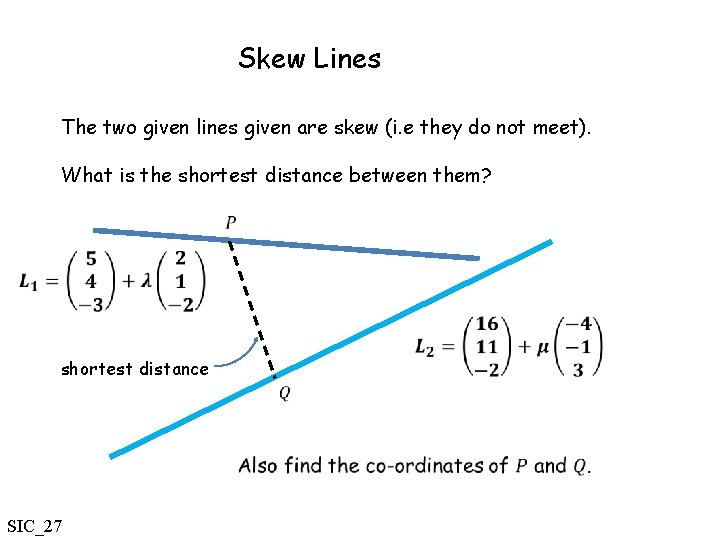Skew Lines The two given lines given are skew (i. e they do not meet). What is the shortest distance between them? shortest distance SIC_27Skew Lines The two given lines given are skew (i. e they do not meet). What is the shortest distance between them? shortest distance SIC_27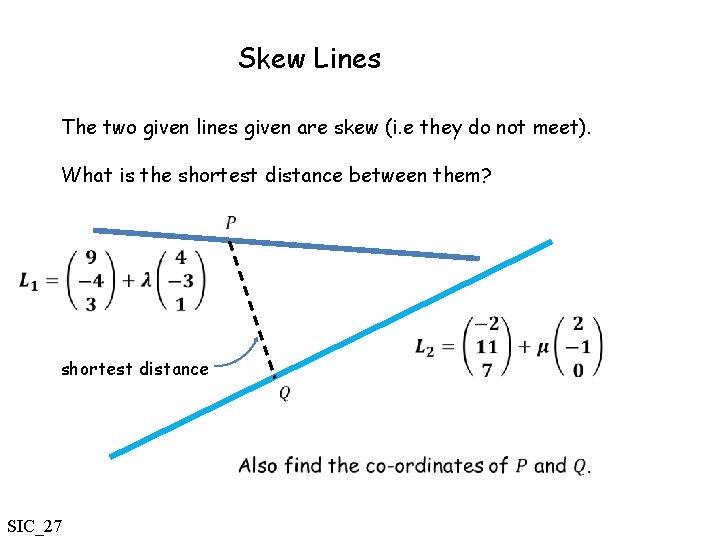Skew Lines The two given lines given are skew (i. e they do not meet). What is the shortest distance between them? shortest distance SIC_27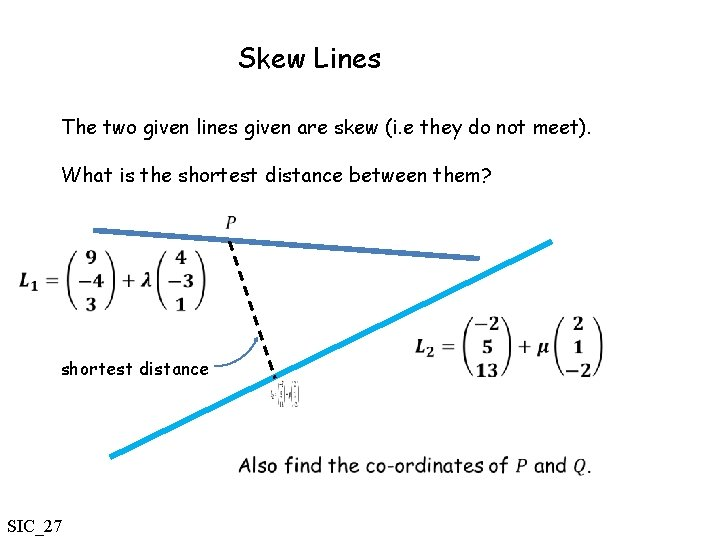Skew Lines The two given lines given are skew (i. e they do not meet). What is the shortest distance between them? shortest distance SIC_27Skew Lines The two given lines given are skew (i. e they do not meet). What is the shortest distance between them? shortest distance SIC_27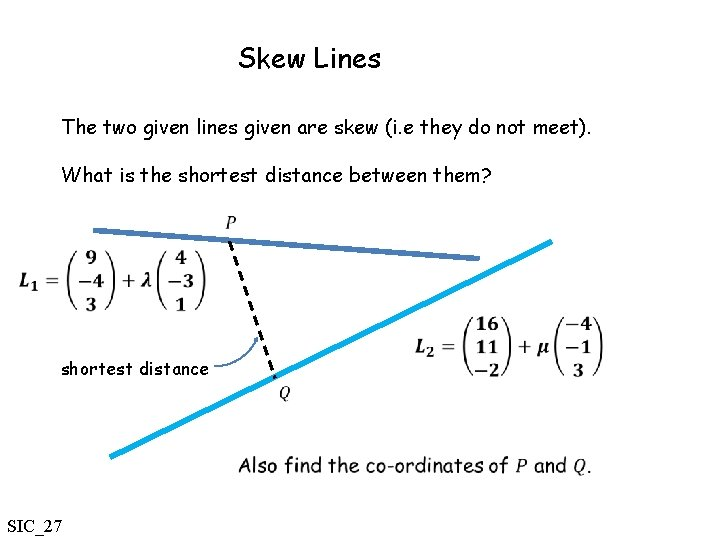Skew Lines The two given lines given are skew (i. e they do not meet). What is the shortest distance between them? shortest distance SIC_27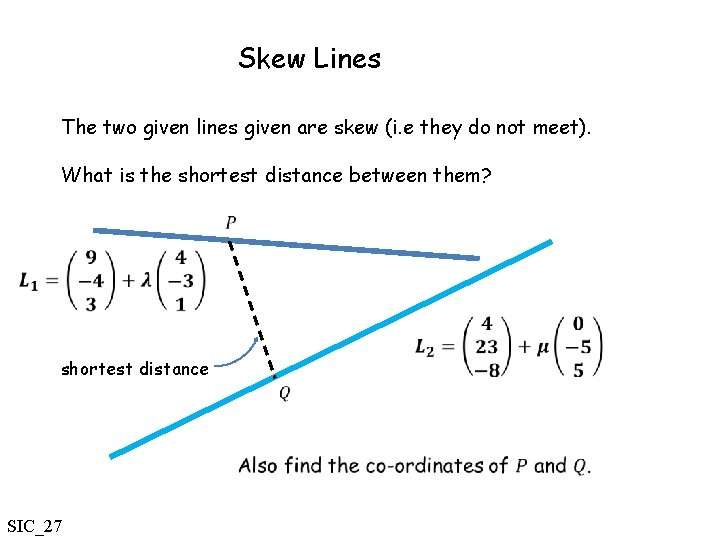Skew Lines The two given lines given are skew (i. e they do not meet). What is the shortest distance between them? shortest distance SIC_27Skew Lines The two given lines given are skew (i. e they do not meet). What is the shortest distance between them? shortest distance SIC_27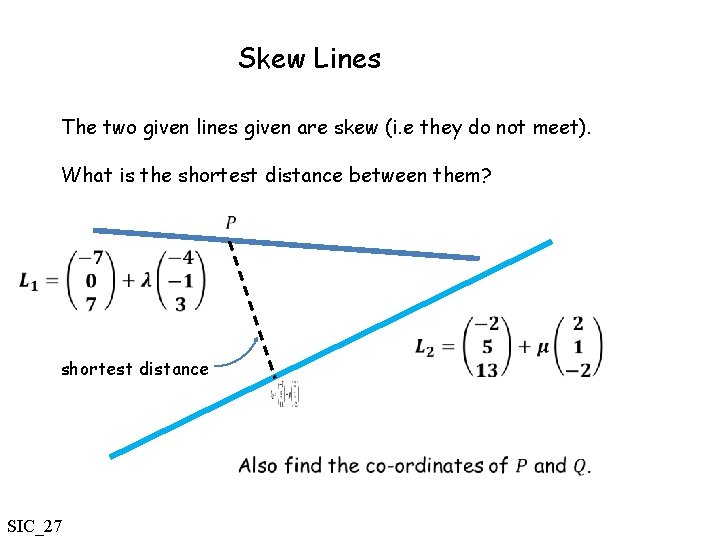Skew Lines The two given lines given are skew (i. e they do not meet). What is the shortest distance between them? shortest distance SIC_27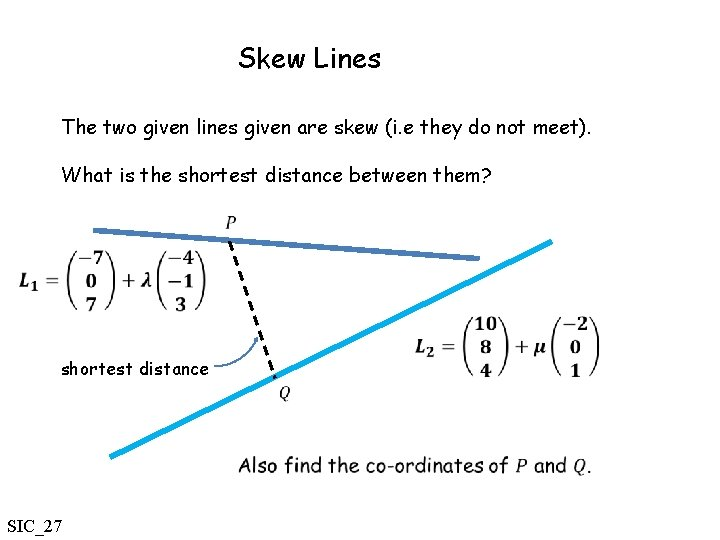Skew Lines The two given lines given are skew (i. e they do not meet). What is the shortest distance between them? shortest distance SIC_27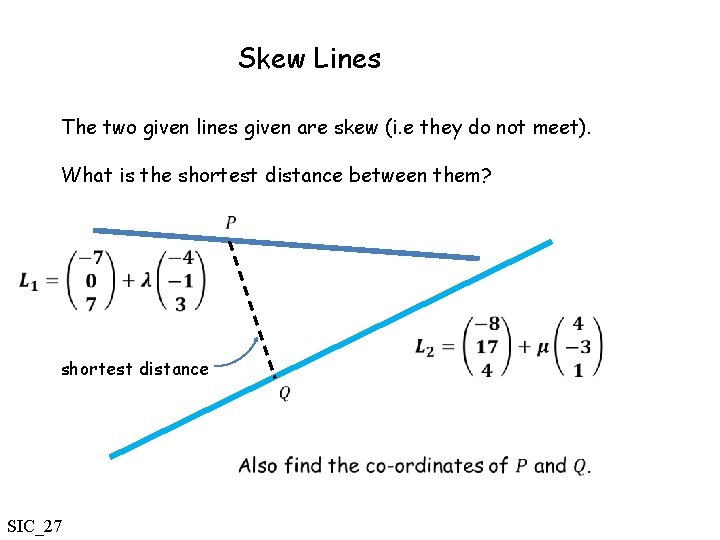Skew Lines The two given lines given are skew (i. e they do not meet). What is the shortest distance between them? shortest distance SIC_27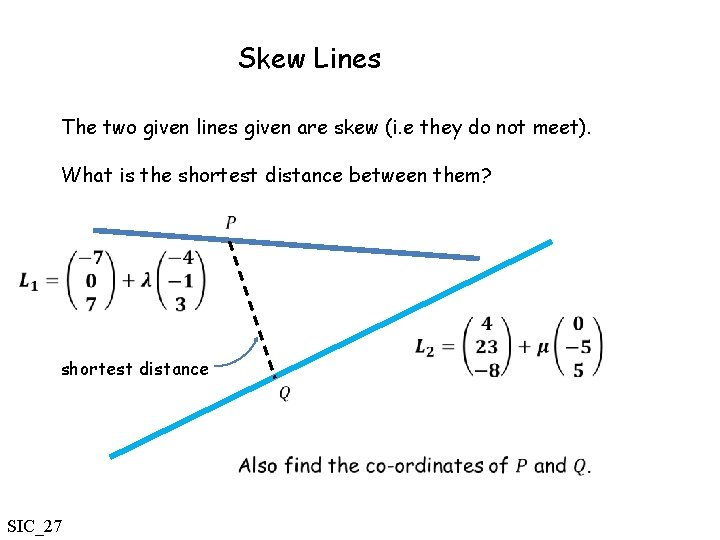Skew Lines The two given lines given are skew (i. e they do not meet). What is the shortest distance between them? shortest distance SIC_27